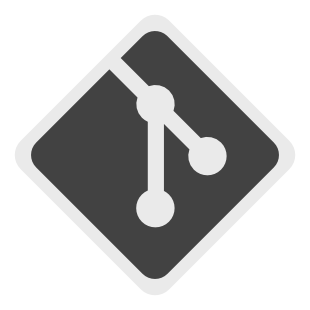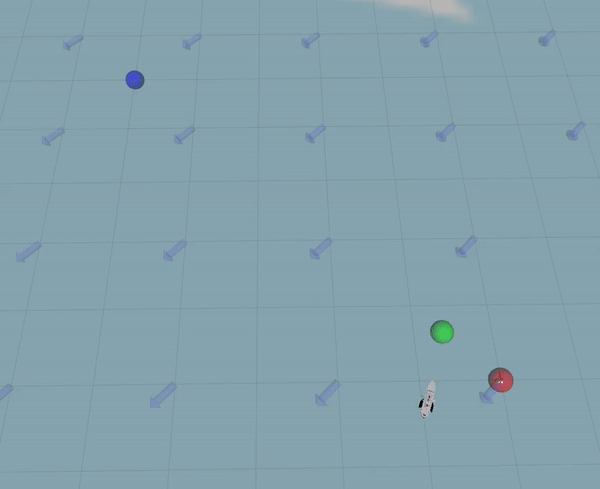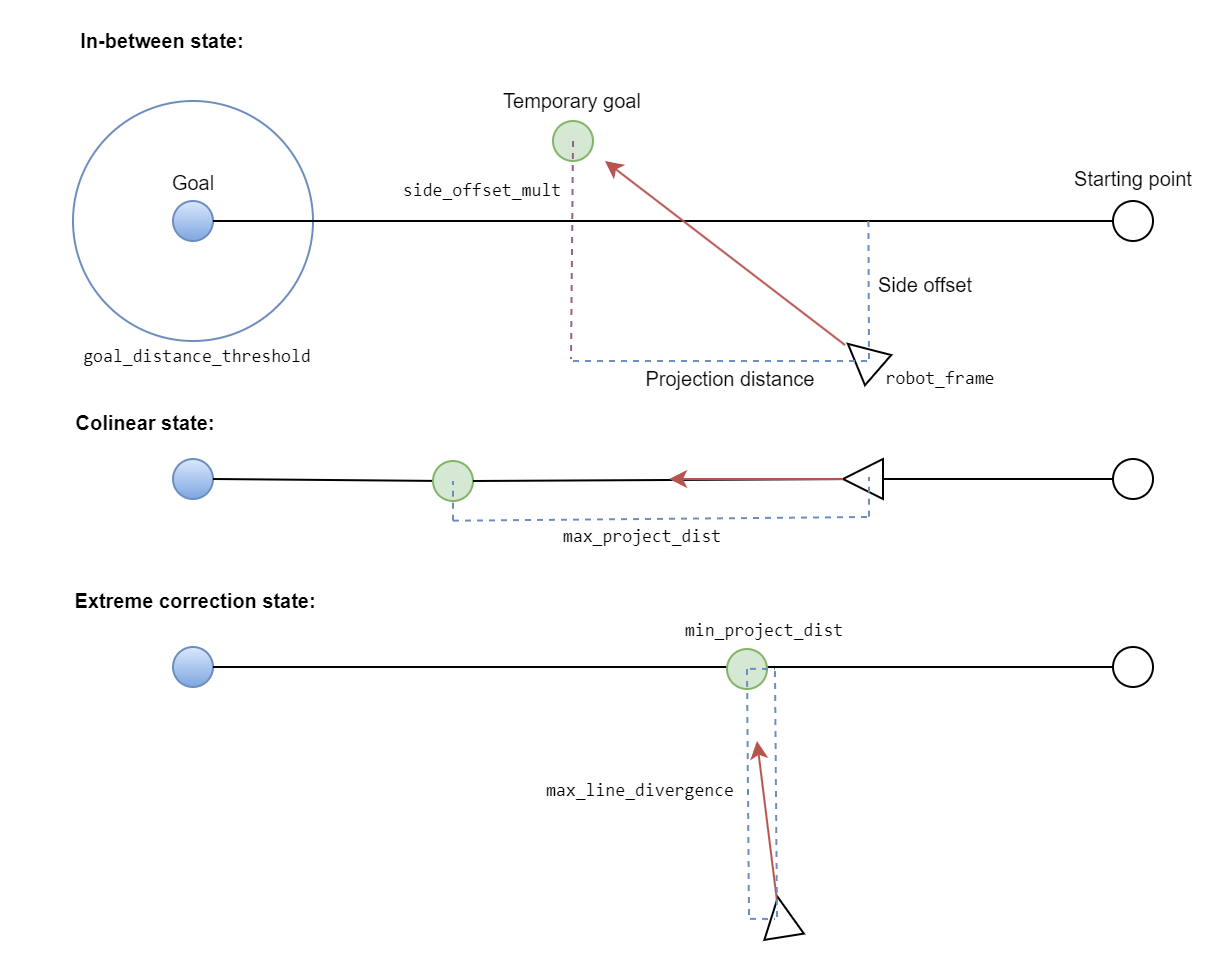### Repository Summary

 Checkout URI https://github.com/MoffKalast/line_planner.git VCS Type git VCS Version noetic-devel Last Updated 2023-06-10 Dev Status MAINTAINED CI status No Continuous Integration Released UNRELEASED Tags No category tags. Contributing Help Wanted (0) Good First Issues (0) Pull Requests to Review (0)

### Packages

Name Version
line_planner 0.0.1

# Simple Projecting Line Planner

A local planner that takes two goals (last and next), and follows a projected goal that keeps it close to the line segment between the two goals. Designed to resist strong side forces, such as from wind or water current.## Params

Note that min speed should be less or equal to max speed.

``````<node name="line_planner" pkg="line_planner" type="line_planner_node.py" output="screen">

<param name="publish_debug_markers" value="true"/>

<param name="max_turning_velocity" value="0.9"/>
<param name="max_linear_velocity" value="0.45"/>

<!-- If base_link is this far away from the line, the projected distance will be min and scale to max when it's on the line.-->
<param name="max_line_divergence" value="1.0"/>

<param name="min_project_dist" value="0.15"/>
<param name="max_project_dist" value="1.2"/>

<!-- Distance at which the goal is considered reached.-->
<param name="goal_distance_threshold" value="0.6"/>

<!-- PID params for heading control.-->
<param name="P" value="3.0"/>
<param name="I" value="0.001"/>
<param name="D" value="65.0"/>

<!-- Update rate, should be about the same as localization rate.-->
<param name="rate" value="30"/>

<!-- If we make no or negative progress for this long, the goal is aborted. (seconds)-->
<param name="abort_timeout" value="30.0"/>

</node>

``````

Here's a diagram showing the possible states of the planner, and which distances each parameter affects:## Subscribed Topics

• `/move_base_simple/goal` (PoseStamped), takes the current position as the starting point and moves towards the goal

• `/move_base_simple/clear` (Empty), stops all movement immediately

• `/move_base_simple/waypoints` (Path), takes each two consecutive points and navigates along the line between them

## Published Topics

• `/cmd_vel` (JointState), publishes velocity for both wheels/tracks/propellers

• `line_planner/active` (Bool), publishes the acitvity state of the planner

• `line_planner/plan` (Path), publishes a nav plan, also the entire route if given

• `line_planner/markers` (MarkerArray), publishes debug markers shown above

## Dynamic Reconfigure Params

• `publish_debug_markers` (bool_t), if set to True, the node will publish markers for debugging purposes.

• `max_turning_velocity` (double_t), the maximum velocity at which the robot can turn.

• `max_linear_velocity` (double_t), the maximum linear velocity of the robot.

• `linear_acceleration` (double_t), the linear acceleration/deceleration of the robot.

• `max_line_divergence` (double_t), the maximum distance that the robot can diverge from the line between the goals.

• `min_project_dist` (double_t), the minimum projection distance for the goal.

• `max_project_dist` (double_t), the maximum projection distance for the goal.

• `goal_distance_threshold` (double_t), the distance at which a goal is considered reached.

• `P` (double_t), the proportional gain for the PID controller that controls the heading of the robot.

• `I` (double_t), the integral gain for the PID controller.

• `D` (double_t), the derivative gain for the PID controller.

• `side_offset_mult` (double_t), multiplier for the side projection of the robot's position.

• `rate` (int_t), the rate at which the robot updates its position and velocity.

Based on the code you've provided, here's an addition to the documentation that describes the functionality of your newly added node:

## Bounding Box to Path Helper

This package also contains a small helper demo node that takes a rectangle defined as a PolygonStamped and turns it into various patterns which then get published as a Path that the line planer can execute. Right now it provides three different patterns: lawnmower, expanding square, and victor sierra.

The lawnmower pattern creates a path in a back-and-forth, or "mowing the lawn" manner. The expanding square pattern creates a square outward spiral path. The victor sierra pattern is a coast guard sector search.

it also accepts a home polygon, which it uses to add a final waypoint that points back home after following the path, so the robot doesn't get stuck out of wifi range.

## Params

``````<node name="area_to_path_node" pkg="line_planner" type="area_to_path_node.py" output="screen">
<param name="step_size" value="2.0"/> <!-- determines the distance between paralel lines -->
</node>

``````

## Subscribed Topics

• `/area_to_path/lawnmower` (PolygonStamped), takes a 4 vertex square and creates a lawnmower path inside it.

• `/area_to_path/expanding_square` (PolygonStamped), takes a 4 vertex square and creates an expanding square (spiral) path inside it.

• `/area_to_path/victor_sierra` (PolygonStamped), takes a 4 vertex square and creates a victor sierra (star) path inside it.

• `/area_to_path/home` (PolygonStamped), takes a 4 vertex square and sets the center point as the home position.

## Published Topics

• `/move_base_simple/waypoints` (Path), publishes the created path.

### CONTRIBUTING

No CONTRIBUTING.md found.